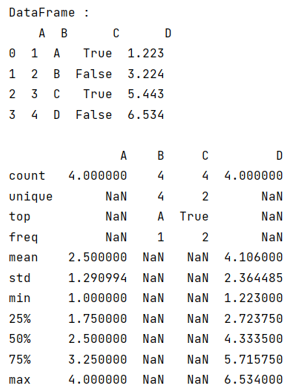# Python - Pandas 'describe' is not returning summary of all columns

Solution of Pandas 'describe' is not returning summary of all columns. By Pranit Sharma Last updated : September 26, 2023

Pandas is a special tool that allows us to perform complex manipulations of data effectively and efficiently. Inside pandas, we mostly deal with a dataset in the form of DataFrame. DataFrames are 2-dimensional data structures in pandas. DataFrames consist of rows, columns, and data.

DataFrame can be created with the help of Python dictionaries or lists but in the real world, CSV files are imported and then converted into DataFrames. Sometimes, DataFrames are first written into CSV files. Here, we are going to write a DataFrame into a CSV file.

While loading a CSV file, it is better that we know about our dataframe in the complete sense, which means we should know about all the data types entered in our dataframe or all the values (faulty or correct) for a better understanding of data and to draw useful insights from the dataframe.

## Fixing: Pandas 'describe' is not returning summary of all columns

Pandas provides us a feature called pandas.DataFrame.describe() which is used to get the summary of all the columns when the dataframe has mixed column types. By default, the behaviour of this method is to only provide a summary for the numerical columns.

When we are dealing with a large dataframe, we may have mixed column types and in that case, we need to pass a parameter as include=all inside describe() method.

Syntax:

```DataFrame.describe(
percentiles=None,
include=None,
exclude=None,
datetime_is_numeric=False
)
```

Let us understand with the help of an example,

## Python Code: Solution for pandas 'describe' is not returning summary of all columns

```# Importing pandas package
import pandas as pd

# Creating a dictionary
d = {
'A':[1,2,3,4],
'B':['A','B','C','D'],
'C':[True,False,True,False],
'D':[1.223,3.224,5.443,6.534]
}

# Creating a dataframe
df = pd.DataFrame(d)

# Display Dataframe
print("DataFrame :\n",df,"\n")

# Using describe method
print(df.describe(include='all'))
```

### Output

The output of the above program is: